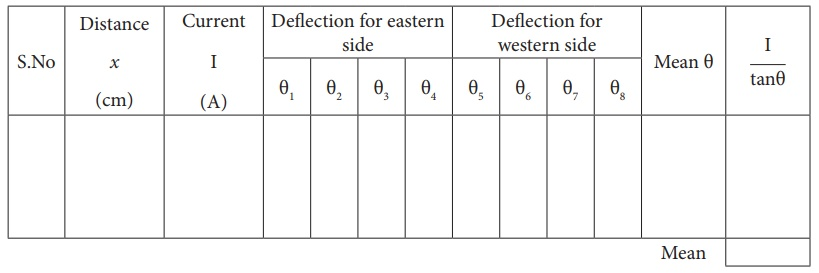Home | | Physics 12th Std | Magnetic Field Along the Axis of a Circular Coil-Determination of BH

# Magnetic Field Along the Axis of a Circular Coil-Determination of BH

To determine the horizontal component of EarthŌĆÖs magnetic field using current carrying circular coil and deflection magnetometer.

MAGNETIC FIELD ALONG THE AXIS OF A CIRCULAR COIL-DETERMINATION OF BH

## AIM

To determine the horizontal component of EarthŌĆÖs magnetic field using current carrying circular coil and deflection magnetometer.

## APPARATUS REQUIRED

Circular coil apparatus, compass box, rheostat, battery or power supply, ammeter, commutator, key and connecting wires.

## FORMULAwhere,

BH ŌåÆ Horizontal component of EarthŌĆÖs magnetic field (T)

┬Ąo ŌåÆ Permeability of the free space (4ŽĆ x 10-7 H m-1)

n ŌåÆ Number of turns included in the circuit (No unit)

I ŌåÆ Current flowing through the coil (A)

r ŌåÆ Radius of the circular coil (m)

x ŌåÆ Distance between center of compass box and centre of the coil (m)

## CIRCUIT DIAGRAM## PROCEDURE

┬Ę        The preliminary adjustments are carried out as follows.

i. The leveling screws are adjusted so that the circular coil is vertical.

ii. The wooden bench is adjusted to be along the magnetic east-west direction i.e., along aluminium pointer.

iii. The circular coil is rotated so that its plane is in magnetic meridian i.e., along the north-south direction.

iv. A compass box is placed with its centre coinciding with the axis of the coil.

v. The compass box alone is rotated till the aluminium pointer reads 0┬░ ŌłÆ 0┬░

┬Ę        Electrical connections are made as shown in the circuit diagram.

┬Ę        The compass box is placed along its axis, with its centre at a distance x from the centre of the coil on one side.

┬Ę        A suitable current (1A) is passed through the coil by adjusting rheostat so that the deflection of the aluminium pointer lies between 30 ┬║ and 60 ┬║.

┬Ę        The value of the current I is noted from ammeter.

┬Ę        Two readings ╬Ė1 and ╬Ė2 corresponding to two ends of the pointer are noted.

┬Ę        Now the direction of the current is reversed using commutator, two more readings ╬Ė3 and ╬Ė4 are noted.

┬Ę        Now the compass box is taken to the other side and is kept at the same distance x.

┬Ę        Four more readings ╬Ė5, ╬Ė6, ╬Ė7 and ╬Ė8 are taken as done before.

┬Ę        These eight readings and their average value are tabulated.

┬Ę        The experiment is repeated for another value of current, say 1.5ŌĆåA by keeping the compass box at the same distance x.

┬Ę        The radius of the circular coil is found by measuring the circumference of the coil using a thread around the coil.

┬Ę        The number of turns n of the coil is noted.

┬Ę        From the values of n, r, x and I/tan╬Ė, the horizontal component of EarthŌĆÖs magnetic field is now found using the formula.

## OBSERVATION

Number of turns in the coil n=

Circumference of the coil (2ŽĆr) =

Radius of the coil r =

To find horizontal component of the EarthŌĆÖs magnetic field## CALCULATION## RESULT

Horizontal component of the EarthŌĆÖs magnetic field at a place = _________T

Tags : Physics Practical Experiment , 12th Physics : Practical
Study Material, Lecturing Notes, Assignment, Reference, Wiki description explanation, brief detail
12th Physics : Practical : Magnetic Field Along the Axis of a Circular Coil-Determination of BH | Physics Practical Experiment